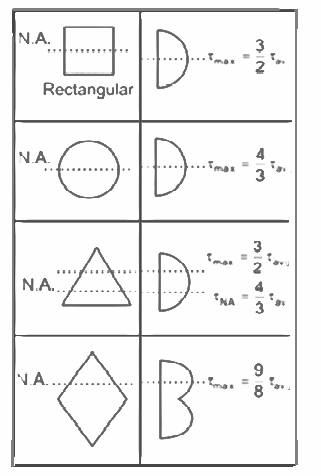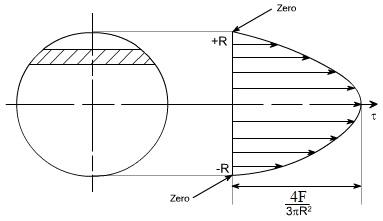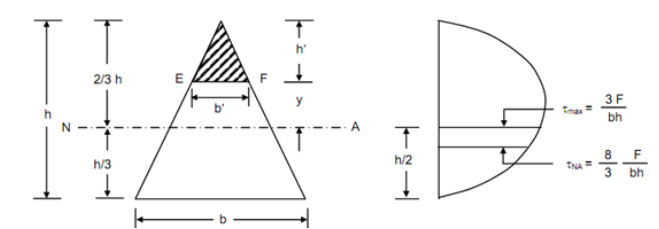Select Page

# Shear Stresses in Beams

Shear Stresses in Beams

Contents

Explain the variation of Shear Stress in the Beams 1

List various assumptions that are taken in the analysis of Shear Stress in the Beams 3

Describe and calculate the Shear Stress Distribution for the Rectangular Section 4

Describe and calculate the Shear Stress Distribution for the Circular Section 5

Describe and calculate the Shear Stress Distribution for the Triangular Section 6

Describe and calculate the Shear Stress Distribution for the Square Section 8

Describe and calculate the Shear Stress Distribution for I-section 9

Describe and calculate the Shear Stress Distribution for T-Section 10

Describe and calculate the Shear Stress Distribution for Channel-Section 11

Explain the concept of Shear Center 12

# Explain the variation of Shear Stress in the Beams

Shear stress is a measure of the internal force within a material that is caused by an external force applied perpendicular to its longitudinal axis. Understanding the variation of shear stress in beams is important for analysing the behaviour and strength of these structures.

In beams, shear stress varies along the cross-section of the beam due to the distribution of internal forces. The maximum shear stress occurs at the top and bottom surfaces of the beam, while the minimum shear stress occurs at the neutral axis. The neutral axis is the point in the cross-section of the beam where the shear stress is equal to zero.

The variation of shear stress in beams can be expressed mathematically using the following equation:

Shear Stress (τ) = Vy / I

Where:

V is the shear force acting on the beam

y is the distance from the neutral axis to the point of interest

I is the moment of inertia of the cross-section of the beam

It is important to note that the above equation is only valid for beams subjected to pure shear loads. In actual engineering practice, more complex analysis is required to determine the variation of shear stress in beams subjected to more complex loading conditions.

In conclusion, the variation of shear stress in beams is an important aspect of mechanical engineering as it affects the behaviour and strength of these structures. Understanding the variation of shear stress allows engineers to design beams that can withstand the applied loads and ensure their safety and functionality.# List various assumptions that are taken in the analysis of Shear Stress in the Beams

Shear stress is a measure of the internal force within a material that is caused by an external force applied perpendicular to its longitudinal axis. Understanding the assumptions that are taken in the analysis of shear stress in beams is important for accurately analysing the behaviour and strength of these structures.

Here are some of the common assumptions taken in the analysis of shear stress in beams:

1. Linear Elastic Material: It is assumed that the material behaviour is linear and elastic. This means that the material exhibits a proportional relationship between stress and strain and returns to its original shape after the loading is removed.
2. Homogeneous Material: It is assumed that the material is homogeneous, i.e., the material properties are constant throughout the cross-section of the beam.
3. Cross-Sectional Shape: It is assumed that the cross-sectional shape of the beam is constant and does not change due to loading.
4. Straight Beams: It is assumed that the beams are straight and do not deform significantly under loading.
5. Transverse Loading: It is assumed that the external loads acting on the beams are transverse in nature, i.e., they are applied perpendicular to the longitudinal axis of the beam.
6. Pure Shear Loading: It is assumed that the beams are subjected to pure shear loading, i.e., the internal forces are only shear forces.
7. Plane Stress: It is assumed that the stresses in the beams are plane stresses, i.e., the stress is constant through the thickness of the beam.

It is important to note that these assumptions are idealised conditions and may not always accurately reflect real-world situations. However, they are commonly used in the analysis of shear stress in beams due to their simplicity and ease of use.

In conclusion, the assumptions taken in the analysis of shear stress in beams play a crucial role in accurately determining the behaviour and strength of these structures. Understanding these assumptions allows engineers to design beams that can withstand the applied loads and ensure their safety and functionality.

# Describe and calculate the Shear Stress Distribution for the Rectangular Section

Shear stress is a measure of the internal force within a material that is caused by an external force applied perpendicular to its longitudinal axis. Understanding the shear stress distribution in a rectangular section is important for analysing the behaviour and strength of structures such as beams.

For a rectangular section, the shear stress distribution can be calculated using the following equation:

Shear Stress (τ) = Vy / I

Where:

V is the shear force acting on the beam

y is the distance from the neutral axis to the point of interest

I is the moment of inertia of the cross-section of the beam

It is important to note that the above equation is only valid for beams subjected to pure shear loads. In actual engineering practice, more complex analysis is required to determine the shear stress distribution in beams subjected to more complex loading conditions.

The shear stress distribution in a rectangular section is uniform across the cross-section, meaning that the shear stress is constant at any given height. The maximum shear stress occurs at the top and bottom surfaces of the beam, while the minimum shear stress occurs at the neutral axis.

In conclusion, understanding the shear stress distribution in a rectangular section is important for accurately analyzing the behaviour and strength of beams and other structures. By knowing the shear stress distribution, engineers can design beams that can withstand the applied loads and ensure their safety and functionality.

# Describe and calculate the Shear Stress Distribution for the Circular Section

Shear stress is a measure of the internal force within a material that is caused by an external force applied perpendicular to its longitudinal axis. Understanding the shear stress distribution in a circular section is important for analyzing the behaviour and strength of structures such as beams.

For a circular section, the shear stress distribution can be calculated using the following equation:

Shear Stress (τ) = V r / 2A

Where:

V is the shear force acting on the beam

r is the radial distance from the center of the circle to the point of interest

A is the cross-sectional area of the circular section

It is important to note that the above equation is only valid for beams subjected to pure shear loads. In actual engineering practice, more complex analysis is required to determine the shear stress distribution in beams subjected to more complex loading conditions.

The shear stress distribution in a circular section is non-uniform, meaning that the shear stress changes with the radial distance from the center of the circle. The maximum shear stress occurs at the outer surface of the beam, while the minimum shear stress occurs at the center of the circle.

In conclusion, understanding the shear stress distribution in a circular section is important for accurately analyzing the behaviour and strength of beams and other structures. By knowing the shear stress distribution, engineers can design beams that can withstand the applied loads and ensure their safety and functionality.# Describe and calculate the Shear Stress Distribution for the Triangular Section

Shear stress is a measure of the internal force within a material that is caused by an external force applied perpendicular to its longitudinal axis. Understanding the shear stress distribution in a triangular section is important for analysing the behaviour and strength of structures such as beams.

For a triangular section, the shear stress distribution can be calculated using the following equation:

Shear Stress (τ) = Vh / 2A

Where:

V is the shear force acting on the beam

h is the height of the triangle at the point of interest

A is the cross-sectional area of the triangular section

It is important to note that the above equation is only valid for beams subjected to pure shear loads. In actual engineering practice, more complex analysis is required to determine the shear stress distribution in beams subjected to more complex loading conditions.

The shear stress distribution in a triangular section is non-uniform, meaning that the shear stress changes with the height of the triangle. The maximum shear stress occurs at the top of the triangle, while the minimum shear stress occurs at the base of the triangle.

In conclusion, understanding the shear stress distribution in a triangular section is important for accurately analyzing the behaviour and strength of beams and other structures. By knowing the shear stress distribution, engineers can design beams that can withstand the applied loads and ensure their safety and functionality.# Describe and calculate the Shear Stress Distribution for the Square Section

Shear stress is a measure of the internal force within a material that is caused by an external force applied perpendicular to its longitudinal axis. Understanding the shear stress distribution in a square section is important for analyzing the behaviour and strength of structures such as beams.

For a square section, the shear stress distribution can be calculated using the following equation:

Shear Stress (τ) = Vy / 2A

Where:

V is the shear force acting on the beam

y is the distance from the center of the square to the point of interest

A is the cross-sectional area of the square section

It is important to note that the above equation is only valid for beams subjected to pure shear loads. In actual engineering practice, more complex analysis is required to determine the shear stress distribution in beams subjected to more complex loading conditions.

The shear stress distribution in a square section is non-uniform, meaning that the shear stress changes with the distance from the center of the square. The maximum shear stress occurs at the corners of the square, while the minimum shear stress occurs at the center of the square.

In conclusion, understanding the shear stress distribution in a square section is important for accurately analyzing the behaviour and strength of beams and other structures. By knowing the shear stress distribution, engineers can design beams that can withstand the applied loads and ensure their safety and functionality.

# Describe and calculate the Shear Stress Distribution for I-section

An I-section, also known as an I-beam or H-beam, is a commonly used beam shape in construction and engineering. Understanding the shear stress distribution in an I-section is important for analysing the behaviour and strength of structures such as beams.

For an I-section, the shear stress distribution can be calculated using the following equation:

Shear Stress (τ) = Vy / 2A

Where:

V is the shear force acting on the beam

y is the distance from the neutral axis to the point of interest

A is the cross-sectional area of the I-section

It is important to note that the above equation is only valid for beams subjected to pure shear loads. In actual engineering practice, more complex analysis is required to determine the shear stress distribution in beams subjected to more complex loading conditions.

The shear stress distribution in an I-section is non-uniform, meaning that the shear stress changes with the distance from the neutral axis. The maximum shear stress occurs at the flanges of the I-section, while the minimum shear stress occurs at the neutral axis.

In conclusion, understanding the shear stress distribution in an I-section is important for accurately analyzing the behaviour and strength of beams and other structures. By knowing the shear stress distribution, engineers can design beams that can withstand the applied loads and ensure their safety and functionality.

# Describe and calculate the Shear Stress Distribution for T-Section

A T-section, also known as a T-beam, is a commonly used beam shape in construction and engineering. Understanding the shear stress distribution in a T-section is important for analysing the behaviour and strength of structures such as beams.

For a T-section, the shear stress distribution can be calculated using the following equation:

Shear Stress (τ) = V y / 2A

Where:

V is the shear force acting on the beam

y is the distance from the neutral axis to the point of interest

A is the cross-sectional area of the T-section

It is important to note that the above equation is only valid for beams subjected to pure shear loads. In actual engineering practice, more complex analysis is required to determine the shear stress distribution in beams subjected to more complex loading conditions.

The shear stress distribution in a T-section is non-uniform, meaning that the shear stress changes with the distance from the neutral axis. The maximum shear stress occurs at the flanges of the T-section, while the minimum shear stress occurs at the neutral axis.

In conclusion, understanding the shear stress distribution in a T-section is important for accurately analyzing the behaviour and strength of beams and other structures. By knowing the shear stress distribution, engineers can design beams that can withstand the applied loads and ensure their safety and functionality.

# Describe and calculate the Shear Stress Distribution for Channel-Section

A channel-section, also known as a C-section or U-section, is a commonly used beam shape in construction and engineering. Understanding the shear stress distribution in a channel-section is important for analyzing the behaviour and strength of structures such as beams.

For a channel-section, the shear stress distribution can be calculated using the following equation:

Shear Stress (τ) = V y / 2A

Where:

V is the shear force acting on the beam

y is the distance from the neutral axis to the point of interest

A is the cross-sectional area of the channel-section

It is important to note that the above equation is only valid for beams subjected to pure shear loads. In actual engineering practice, more complex analysis is required to determine the shear stress distribution in beams subjected to more complex loading conditions.

The shear stress distribution in a channel-section is non-uniform, meaning that the shear stress changes with the distance from the neutral axis. The maximum shear stress occurs at the flanges of the channel-section, while the minimum shear stress occurs at the neutral axis.

In conclusion, understanding the shear stress distribution in a channel-section is important for accurately analyzing the behaviour and strength of beams and other structures. By knowing the shear stress distribution, engineers can design beams that can withstand the applied loads and ensure their safety and functionality.

# Explain the concept of Shear Center

The concept of the shear center is an important aspect of structural analysis, particularly for beams and other structures subjected to shear loads. The shear center is defined as the point at which the shear stress distribution in a structure is uniform, meaning that the shear stress does not change along the length of the structure.

The shear center is important because it helps engineers understand the behaviour of beams and other structures under shear loads. For example, if a beam is subjected to a shear load, the shear stress will be highest at the points farthest from the shear center and lowest at the shear center itself. This information can be used to design beams that can withstand the applied loads and ensure their safety and functionality.

The shear center is typically determined using advanced analytical methods, such as the application of mechanics equations or finite element analysis. In some cases, the shear center may be determined experimentally, by testing a beam under load and measuring the resulting deflection and shear stress distribution.

It is important to note that the shear center is not always located at the geometric center of a structure. For some beam shapes, such as rectangular or circular sections, the shear center coincides with the geometric center. However, for more complex shapes, such as I-sections or T-sections, the shear center may be located elsewhere.

In conclusion, the concept of the shear center is an important aspect of structural analysis that helps engineers understand the behaviour of beams and other structures under shear loads. By knowing the location of the shear center, engineers can design beams that can withstand the applied loads and ensure their safety and functionality.